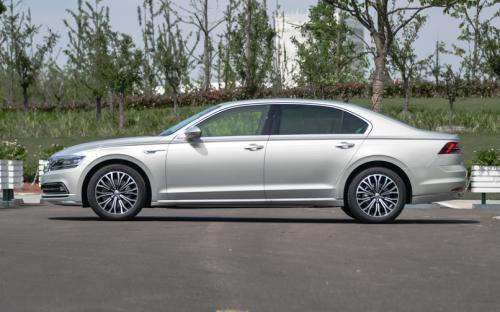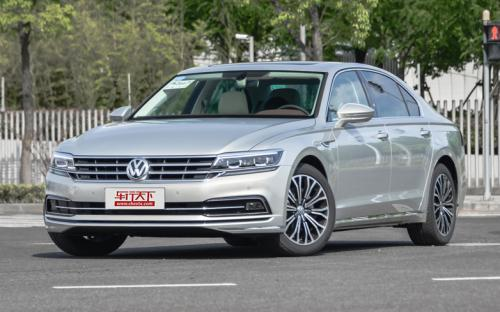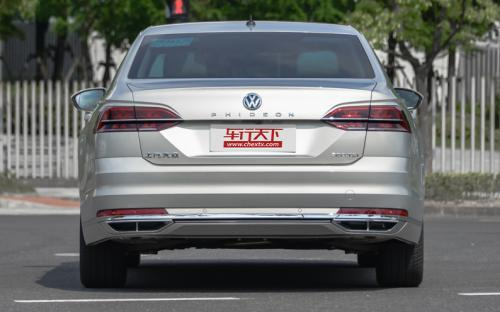## 上海大众 辉昂0 种颜色可选2016款最低售价：34.90 万元起

5074(mm)1893(mm)1489(mm)##### 配置亮点：
• 胎压监测装置

• ISOFIX儿童座椅接口

• 车身稳定控制(ESC/ESP/DSC等)

• 电动天窗

• 定速巡航

• 后倒车雷达

• 真皮座椅

• GPS导航系统

• 氙气大灯

• 后视镜加热

2016款 380TSI 两驱至尊版 (83张)
• 2016款 380TSI 两驱至尊版 (83张)
• 2016款 480 V6 四驱行政旗舰版 (393张)上海大众 辉昂 在售车型

排量 车型 厂商指导价 本地最低报价 购车工具
2.0T 380TSI 两驱御尊版 7挡双离合 34.90万 询底价+对比
380TSI 两驱御尊豪华版 7挡双离合 38.90万 询底价+对比
380TSI 两驱至尊版 7挡双离合 40.90万 询底价+对比
380TSI 两驱行政版 7挡双离合 46.90万 询底价+对比
380TSI 两驱行政旗舰版 7挡双离合 53.90万 询底价+对比
480 V6 四驱至尊版 7挡双离合 52.90万 询底价+对比
480 V6 四驱行政版 7挡双离合 58.90万 询底价+对比
480 V6 四驱行政旗舰版 7挡双离合 65.90万 询底价+对比
3.0T 380TSI 两驱御尊版 7挡双离合 34.90万 询底价+对比
380TSI 两驱御尊豪华版 7挡双离合 38.90万 询底价+对比
380TSI 两驱至尊版 7挡双离合 40.90万 询底价+对比
380TSI 两驱行政版 7挡双离合 46.90万 询底价+对比
380TSI 两驱行政旗舰版 7挡双离合 53.90万 询底价+对比
480 V6 四驱至尊版 7挡双离合 52.90万 询底价+对比
480 V6 四驱行政版 7挡双离合 58.90万 询底价+对比
480 V6 四驱行政旗舰版 7挡双离合 65.90万 询底价+对比

上海大众 辉昂 经销商

查看更多 >>
更多 >>

本地降价排名

### 上海大众 辉昂 动力加速

辉昂 0-100公里加速时间分布在 6.3-8.1秒 属于 高性能级

动力级别 加速时间 车型
运动级(1款) 8.1s 380TSI 两驱御尊版 380TSI 两驱御尊豪华版380TSI 两驱至尊版380TSI 两驱行政版380TSI 两驱行政旗舰版
高性能级(1款) 6.3s 480 V6 四驱至尊版480 V6 四驱行政版480 V6 四驱行政旗舰版

上海大众 辉昂 视频

上海大众 辉昂 新闻资讯

# 上汽大众辉昂正式上市 售价34.90万元起

国产新车 超过9648次关注

2016年10月21日，备受瞩目的上汽大众辉昂PHIDEON正式上市发售。据悉，定位于中大型豪华轿车的辉昂在分别搭载2.0T涡轮增压发动机及3.0TV6机械增压发动机基础上根据配...

# 旗舰升级 上汽大众辉昂或于十月上市

国产新车 超过9545次关注

日前，我们从官方获悉，上汽大众全新中大型车辉昂将于今年10月份正式上市。新车已在北京车展前夕的大众之夜上亮相，动力上将搭载3.0T或者2.0T发动机，提供前驱或者...

猜你喜欢

﻿
• 快速找车
• 选择品牌
• 选择品牌
• A  奥迪
• A  阿斯顿·马丁
• A  阿尔法·罗密欧
• B  宝沃
• B  布加迪
• B  巴博斯
• B  保时捷
• B  宾利
• B  奔驰
• B  宝马
• B  本田
• B  别克
• B  标致
• B  比亚迪
• B  宝骏
• B  北汽制造
• B  北汽新能源
• B  北汽幻速
• B  北汽威旺
• B  北京汽车
• B  奔腾
• B  北汽绅宝
• C  长安
• C  长安商用
• C  长城
• C  昌河
• D  大众
• D  道奇
• D  DS
• D  东南
• D  东风风神
• D  东风风行
• D  东风小康
• D  东风风度
• D  东风
• F  福特
• F  丰田
• F  菲亚特
• F  法拉利
• F  福田
• F  福迪
• F  福汽启腾
• G  观致
• G  广汽传祺
• G  广汽吉奥
• G  GMC
• H  红旗
• H  汉腾汽车
• H  哈弗
• H  哈飞
• H  海格
• H  海马
• H  华颂
• H  黄海
• H  华泰
• H  恒天
• J  吉利汽车
• J  捷豹
• J  Jeep
• J  江淮
• J  江铃
• J  金杯
• J  九龙
• J  金旅
• K  凯翼
• K  凯迪拉克
• K  克莱斯勒
• K  科尼塞克
• K  卡威
• K  开瑞
• L  路虎
• L  林肯
• L  劳斯莱斯
• L  兰博基尼
• L  雷克萨斯
• L  铃木
• L  雷诺
• L  理念
• L  力帆
• L  莲花汽车
• L  猎豹
• L  路特斯
• L  陆风
• M  马自达
• M  MG
• M  MINI
• M  玛莎拉蒂
• M  摩根
• M  迈凯轮
• N  纳智捷
• O  欧宝
• O  讴歌
• O  欧朗
• Q  奇瑞
• Q  起亚
• Q  启辰
• R  日产
• R  荣威
• R  瑞麒
• S  三菱
• S  斯威汽车
• S  萨博
• S  smart
• S  斯柯达
• S  斯巴鲁
• S  思铭
• S  双龙
• S  上汽大通
• S  双环
• T  特斯拉
• T  腾势
• W  沃尔沃
• W  五菱汽车
• W  五十铃
• W  威兹曼
• W  威麟
• X  现代
• X  雪佛兰
• X  雪铁龙
• X  西雅特
• Y  一汽
• Y  英菲尼迪
• Y  英致
• Y  依维柯
• Y  野马汽车
• Y  永源
• Z  众泰
• Z  中华
• Z  中兴
• Z  知豆
• 选择车系
• 选择车系
• 车型对比
• 选择品牌
• 选择品牌
• A  奥迪
• A  阿斯顿·马丁
• A  阿尔法·罗密欧
• B  宝沃
• B  布加迪
• B  巴博斯
• B  保时捷
• B  宾利
• B  奔驰
• B  宝马
• B  本田
• B  别克
• B  标致
• B  比亚迪
• B  宝骏
• B  北汽制造
• B  北汽新能源
• B  北汽幻速
• B  北汽威旺
• B  北京汽车
• B  奔腾
• B  北汽绅宝
• C  长安
• C  长安商用
• C  长城
• C  昌河
• D  大众
• D  道奇
• D  DS
• D  东南
• D  东风风神
• D  东风风行
• D  东风小康
• D  东风风度
• D  东风
• F  福特
• F  丰田
• F  菲亚特
• F  法拉利
• F  福田
• F  福迪
• F  福汽启腾
• G  观致
• G  广汽传祺
• G  广汽吉奥
• G  GMC
• H  红旗
• H  汉腾汽车
• H  哈弗
• H  哈飞
• H  海格
• H  海马
• H  华颂
• H  黄海
• H  华泰
• H  恒天
• J  吉利汽车
• J  捷豹
• J  Jeep
• J  江淮
• J  江铃
• J  金杯
• J  九龙
• J  金旅
• K  凯翼
• K  凯迪拉克
• K  克莱斯勒
• K  科尼塞克
• K  卡威
• K  开瑞
• L  路虎
• L  林肯
• L  劳斯莱斯
• L  兰博基尼
• L  雷克萨斯
• L  铃木
• L  雷诺
• L  理念
• L  力帆
• L  莲花汽车
• L  猎豹
• L  路特斯
• L  陆风
• M  马自达
• M  MG
• M  MINI
• M  玛莎拉蒂
• M  摩根
• M  迈凯轮
• N  纳智捷
• O  欧宝
• O  讴歌
• O  欧朗
• Q  奇瑞
• Q  起亚
• Q  启辰
• R  日产
• R  荣威
• R  瑞麒
• S  三菱
• S  斯威汽车
• S  萨博
• S  smart
• S  斯柯达
• S  斯巴鲁
• S  思铭
• S  双龙
• S  上汽大通
• S  双环
• T  特斯拉
• T  腾势
• W  沃尔沃
• W  五菱汽车
• W  五十铃
• W  威兹曼
• W  威麟
• X  现代
• X  雪佛兰
• X  雪铁龙
• X  西雅特
• Y  一汽
• Y  英菲尼迪
• Y  英致
• Y  依维柯
• Y  野马汽车
• Y  永源
• Z  众泰
• Z  中华
• Z  中兴
• Z  知豆
• 选择车系
• 选择车系
• 选择车型
• 选择车型
• 意见反馈